5th July, 2022

# Setting up PIL and aggdraw

I've recently been getting into generative art again. This time, I've decided to work with PIL since I've gotten super comfortable with python recently.

The best thing about python for generative art is using the Jupyter notebook - I think its structure works beautifully like a sketchbook.

## Starting with PIL(Pillow)

``````#!conda install pillow -y
## OR
!pip install pillow
``````
```Requirement already satisfied: pillow in /usr/lib/python3/dist-packages (7.0.0)
```

Once pillow is installed, I can import PIL and start using it. To begin with, I define some functions to make it easier to use aggdraw.

``````from PIL import Image, ImageDraw
import random

# Initialize Parameters
image_height = 150
image_width = 900
seed = 58

random.seed(seed)

def getRandomPoint(xoffset = 0):
return (random.randrange(image_height) + xoffset), random.randrange(image_height)

def collapse(arr):
'''
Converts a 2d array into a 1d array
[[1,2,3],[4,5,6]] -> [1,2,3,4,5,6]
'''
return [item for t in arr for item in t]

def getRandomPoints(count, offset = 0):
v = [getRandomPoint(offset) for _ in range(count)]
v = collapse(v)
return v

``````

Once I do that, I can initialize a new image and start drawing. I'm still using only the basic draw functions from PIL.

``````image = Image.new("RGB", (image_width, image_height), "black")
draw = ImageDraw.Draw(image)

draw.polygon([getRandomPoint() for _ in range(3)], fill="green")
draw.polygon([getRandomPoint() for _ in range(5)],
outline="white")
image
``````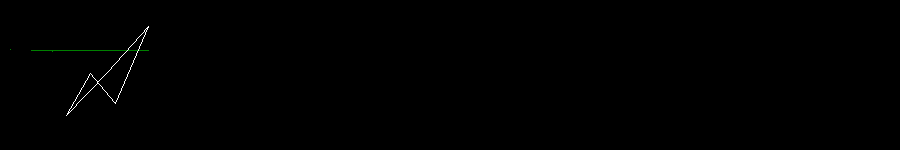## Using the `aggdraw` library

The AggDraw library provides a python interface on top of the AGG library. Anti-Grain Geometry (AGG) is an Open Source, 2D vector graphics library, written by Maxim Shemanarev in C++.

It can be installed using `conda` or `pip` and imported as below

``````#!conda install -c conda-forge aggdraw -y
## OR
!pip install aggdraw

import aggdraw
``````
```Requirement already satisfied: aggdraw in /home/addy/.local/lib/python3.8/site-packages (1.3.15)
```

## The `Draw` Class

• `Draw(image_or_mode, size, color=None)` - (constructor) Creates a drawing interface object.
• Parameters
• `image_or_mode` - A PIL Image, or a mode string. The following modes are supported: “L”, “RGB”, “RGBA”, “BGR”, “BGRA”.
• `size` - If a mode string was given, this argument gives the image size, as a 2-tuple.
• `color` - An optional background color specifier. If a mode string was given, this is used to initialize the image memory. If omitted, it defaults to white with full alpha.

Initializing a `draw` object gives us access to all its drawing methods (`arc`, `chord`, `ellipse`, `line`, `path`, `pieslice`, `polygon`, `rectangle`, `symbol` and `text`). Lets start by looking at `rectangle`:

### `Draw.rectangle(xy, pen, brush)`

• Draws a rectangle. If a brush is given, it is used to fill the rectangle. If a pen is given, it is used to draw an outline around the rectangle. Either one (or both) can be left out.
• Parameters:
• `xy` - A 4-element Python sequence (x, y, x, y), with the upper left corner given first.
• `pen` - Optional pen object created by the Pen factory.
• `brush` - Optional brush created by the Brush factory.

After calling any of the drawing methods, calling `flush()` will actually make the drawings onto the image.

``````offset = 50
d = aggdraw.Draw(image)
v = getRandomPoints(2, offset)
print(v)
d.rectangle(v)
d.flush()
image
``````
```[53, 125, 104, 120]
```That didn't draw anything at all! Thats because all drawing methods also expect either a `Pen` or a `Brush` object as parameters and if you do not supply any one of them, it will not do anything.

## The `Pen` Class

• `Pen(color, width=1, opacity=255)` - (constructor) Creates a pen object.
• Parameters:
• `color` - Pen color. This can be a color tuple, a CSS-style color name, or a color integer (0xaarrggbb).
• `width=` - Optional pen width.
• `opacity=` - Optional pen opacity. The default is to create a solid pen.
• Returns:
• A pen object.

Lets try drawing a rectangle again using this.

``````p = aggdraw.Pen("cyan")
d.rectangle(v, p)
d.flush()
image
``````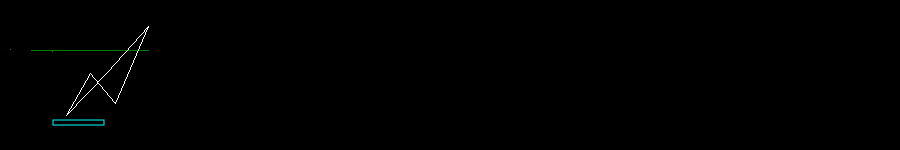## The `Brush` class

• `Brush(color, opacity=255)` - (constructor) Creates a brush object.
• Parameters:
• `color` - Brush color. This can be a color tuple, a CSS-style color name, or a color integer (0xaarrggbb).
• `opacity=` - Optional brush opacity. The default is to create a solid brush.
• Returns:
• A brush object.
``````offset += 50
v = getRandomPoints(2, offset)
b = aggdraw.Brush("green")
d.rectangle(v, b)
d.flush()
image
``````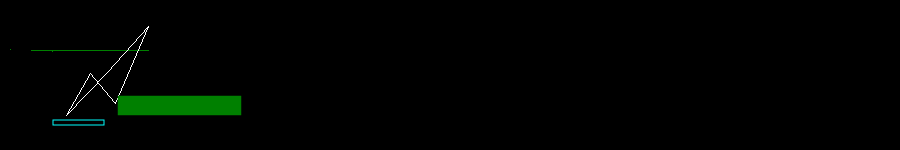### `Draw.line(xy, pen)`

• Draws a line joining all the points on the `xy` parameter.
• Parameters
• `xy` - A Python sequence (x, y, x, y, …). If more than two coordinate pairs are given, they are connected in order, to form a polyline.
• `pen` - A pen object created by the Pen factory method.

Since we are using the same `v` as the rectangle, this function simply draws a diagonal of the rectangle (its inverted because the rectangle vertices themselves are inverted).

``````d.line(v, p)
d.flush()
image
``````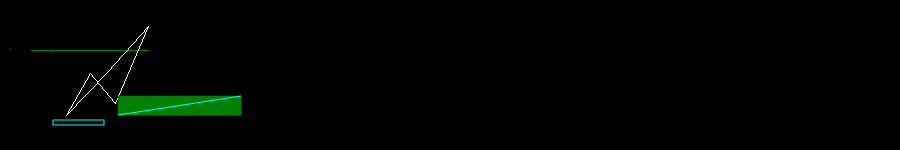### `Draw.arc(xy, start, end, pen=None)`

• Draws an arc.
• Parameters:
• `xy` - A 4-element Python s|equence (x, y, x, y), with the upper left corner given first.
• `start` - Start angle.
• `end` - End angle.
• `pen` - Optional pen object created by the Pen factory.
``````offset += 50
v = getRandomPoints(2, offset)
start = random.randrange(360)
end = random.randrange(360)
d.arc(v, start, end, p)
d.flush()
image
``````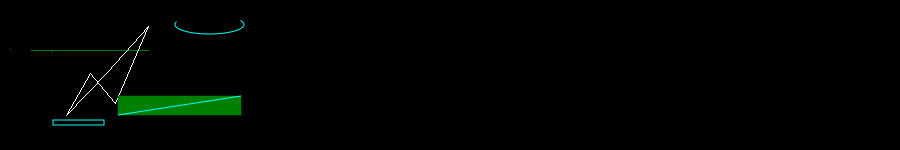### `Draw.chord(xy, start, end, pen=None, brush=None)`

• Draws a chord. If a brush is given, it is used to fill the chord. If a pen is given, it is used to draw an outline around the chord. Either one (or both) can be left out.
• Parameters:
• `xy` : A 4-element Python sequence (x, y, x, y), with the upper left corner given first.
• `start` : Start angle.
• `end` : End angle.
• `pen` : Optional pen object created by the Pen factory.
• `brush`: Optional brush object created by the Brush factory.
``````offset += 50
v = getRandomPoints(2, offset)
start = random.randrange(360)
end = random.randrange(360)
d.chord(v, start, end, p)
d.flush()
image
``````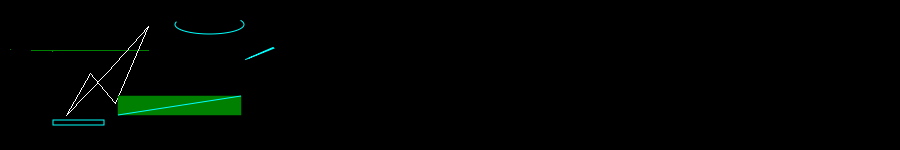### `Draw.ellipse(xy, pen, brush)`

• Draws an ellipse. If a brush is given, it is used to fill the ellipse. If a pen is given, it is used to draw an outline around the ellipse. Either one (or both) can be left out.
• Parameters:
• `xy` - A bounding rectangle, given as a 4-element Python sequence (x, y, x, y), with the upper left corner given first. To draw a circle, make sure the coordinates form a square.
• `pen` - Optional pen object created by the Pen factory.
• `brush` - Optional brush created by the Brush factory.
``````offset += 50
v = getRandomPoints(2, offset)
d.ellipse(v, p)
d.flush()
image
``````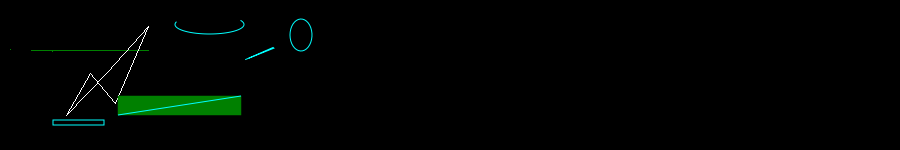### `Draw.pieslice(xy, start, end, pen=None, brush=None)`

• Draws a pieslice. If a brush is given, it is used to fill the pieslice. If a pen is given, it is used to draw an outline around the pieslice. Either one (or both) can be left out.
• Parameters:
• `xy` - A 4-element Python sequence (x, y, x, y), with the upper left corner given first.
• `start` - Start angle.
• `end` - End angle.
• `pen` - Optional pen object created by the Pen factory.
• `brush` - Optional brush object created by the Brush factory.
``````offset += 50
v = getRandomPoints(2, offset)
start = random.randrange(360)
end = random.randrange(360)
d.pieslice(v, start, end, p)
d.flush()
image
``````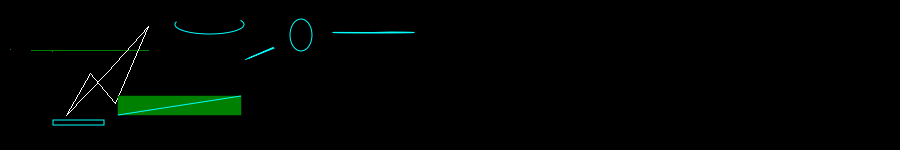### `Draw.polygon(xy, pen, brush)`

• Draws a polygon. If a brush is given, it is used to fill the polygon. If a pen is given, it is used to draw an outline around the polygon. Either one (or both) can be left out.
• Parameters:
• `xy` - A Python sequence (x, y, x, y, …).
• `pen` - Optional pen object created by the Pen factory.
• `brush` - Optional brush object created by the Brush factory.
``````offset += 50
v = getRandomPoints(8, offset)
d.polygon(v, p)
d.flush()
image
``````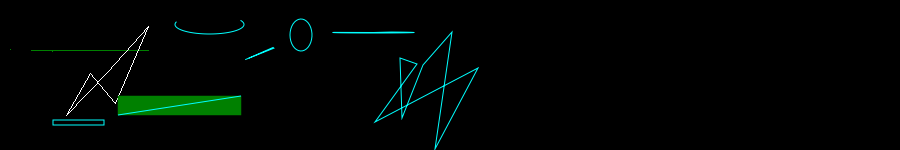## The `Path` Object

• `Path()` - (constructor) creates a path object for use with path.
• Returns:
• A `Path` object.

### `Path.lineto(x, y)`

• Adds a line segment to the path.
• Parameters:
• `x` - The x coordinate
• `y` - The y coordinate

### `Path.close()`

• Closes the current path.

Finally, we need to draw this path on the canvas.

### `Draw.path(xy, path, pen=None, brush=None)`

• Draws a path at the given positions. If a brush is given, it is used to fill the path. If a pen is given, it is used to draw an outline around the path. Either one (or both) can be left out.
• Parameters:
• `xy` - A Python sequence (x, y, x, y, …).
• `path` - A symbol object created by the Path factory.
• `pen` - Optional pen object created by the Pen factory.
• `brush` - Optional brush object created by the Brush factory.
``````offset += 50
path = aggdraw.Path()
path.lineto(*getRandomPoint(offset)) #First .lineto() sets starting point of the path
path.lineto(*getRandomPoint(offset))
path.lineto(*getRandomPoint(offset))
path.lineto(*getRandomPoint(offset))
path.lineto(*getRandomPoint(offset))
path.lineto(*getRandomPoint(offset))
path.close() # Optional in this case
d.path(path, b)
d.flush()
image
``````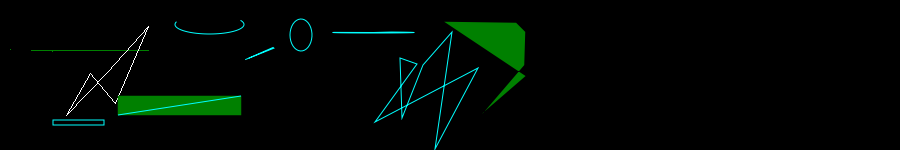### `Path.rlineto(x, y)`

• Adds a line segment to the path, using relative coordinates.
• Parameters:
• `x` - The relative x coordinate
• `y` - The relative y coordinate
``````offset += 50
path = aggdraw.Path()
path.lineto(*getRandomPoint(offset)) #First .lineto() sets starting point of the path
path.rlineto(20, 50)
path.rlineto(-40, -30)
path.rlineto(50, 0)
path.rlineto(-40, 30)
path.close() # Optional in this case
d.path(path, p)
d.flush()
image
``````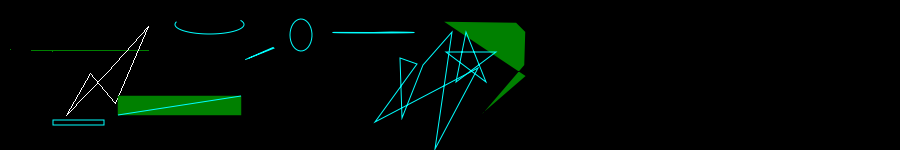### `Path.curveto(x1, y1, x2, y2, x, y)`

• Adds a bezier curve segment to the path.
• Parameters:
• `x1` - The absolute x-coordinate for P1 control point
• `y1` - The absolute y-coordinate
• `x2` - The absolute x-coordinate for P2 control point
• `y2` - The absolute y-coordinate
• `x` - The absolute x-coordinate for P3 control point
• `y` - The absolute y-coordinate

Below, I first draw the control point lines (P0-P1 and P2-P3) then use `curveto` to draw the actual bezier curve.

``````offset += 100
pgrey = aggdraw.Pen("grey")

path = aggdraw.Path()
xs, ys = getRandomPoint(offset)
path.lineto(xs, ys) #First .lineto() sets starting point of the path

x1, y1, x2, y2, xd, yd = getRandomPoints(3, offset)
d.line((xs, ys, x1, y1), pgrey)
d.line((x2, y2, xd, yd), pgrey)
path.curveto(x1, y1, x2, y2, xd, yd)

xs, ys = xd, yd
x1, y1, x2, y2, xd, yd = getRandomPoints(3, offset)
d.line((xs, ys, x1, y1), pgrey)
d.line((x2, y2, xd, yd), pgrey)
path.curveto(x1, y1, x2, y2, xd, yd)

xs, ys = xd, yd
x1, y1, x2, y2, xd, yd = getRandomPoints(3, offset)
d.line((xs, ys, x1, y1), pgrey)
d.line((x2, y2, xd, yd), pgrey)
path.curveto(x1, y1, x2, y2, xd, yd)

xs, ys = xd, yd
x1, y1, x2, y2, xd, yd = getRandomPoints(3, offset)
d.line((xs, ys, x1, y1), pgrey)
d.line((x2, y2, xd, yd), pgrey)
path.curveto(x1, y1, x2, y2, xd, yd)

path.close() # Optional in this case
d.path(path, p)
d.flush()
image
``````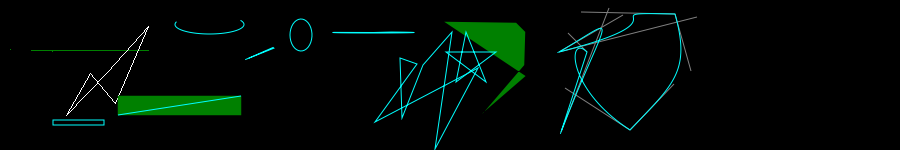### `Path.rcurveto(x1, y1, x2, y2, x, y)`

• Adds a bezier curve segment to the path using relative coordinates.
• Parameters:
• `x1` - The relative x-coordinate for P1 control point
• `y1` - The relative y-coordinate
• `x2` - The relative x-coordinate for P2 control point
• `y2` - The relative y-coordinate
• `x` - The relative x-coordinate for P3 control point
• `y` - The relative y-coordinate

Below, I first draw the control point lines (P0-P1 and P2-P3) in grey then use `rcurveto` to draw the actual bezier curve in blue.

``````offset += 150
pgrey = aggdraw.Pen("grey")

path = aggdraw.Path()
xs, ys = offset, 10
path.lineto(xs, ys) #First .lineto() sets starting point of the path

d.line((xs, ys, xs+40, ys+40), pgrey)
d.line((xs+60, ys+40, xs+100, ys), pgrey)
path.rcurveto(50, 50, 50, 50, 100, 0)

d.line((xs+100, ys, xs+140, ys+40), pgrey)
d.line((xs+60, ys+40, xs + 100, ys + 100), pgrey)
path.rcurveto(50, 50, -50, 50, 0, 100)

d.line(( xs + 100, ys + 100, xs + 140, ys + 140), pgrey)
d.line((xs+40, ys+140, xs, ys), pgrey)
path.rcurveto(50, 50, -50, 50, -100, -100)

path.close() # Optional in this case
d.path(path, p)
d.flush()
image
``````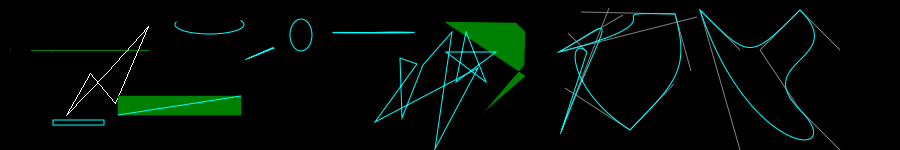And that covers most of the api.